#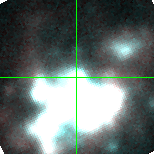M33C-21192 (J013432.74+304709.6)

## Target Notes### Classification: Of/late-WN

(Candidate LBV) On the edge of massive HII region NGC 604. The target is at least 1 magnitude brighter than but unresolved from the 8 other LGGS stars within 2 arcseconds. Measurements are marked "B" by default and marked "X" if there are additional problems with the PSF fit.

## Plots of Brightness and Color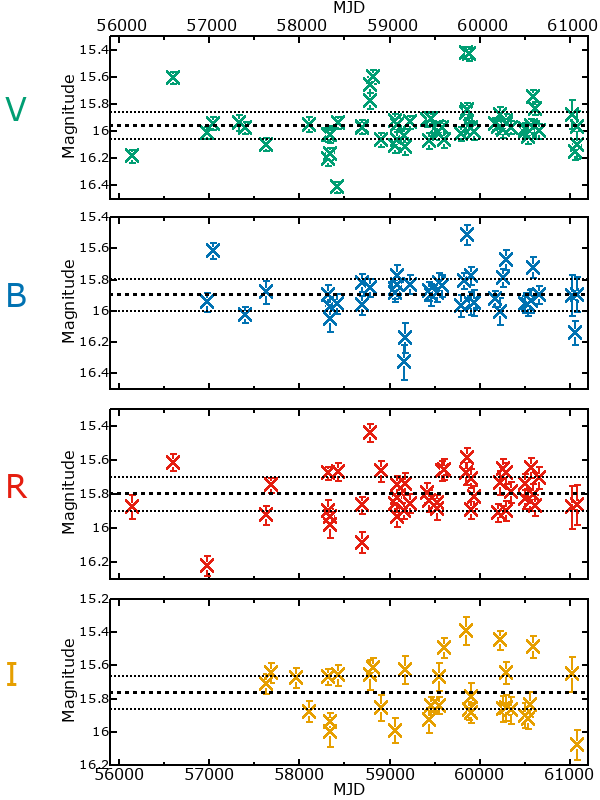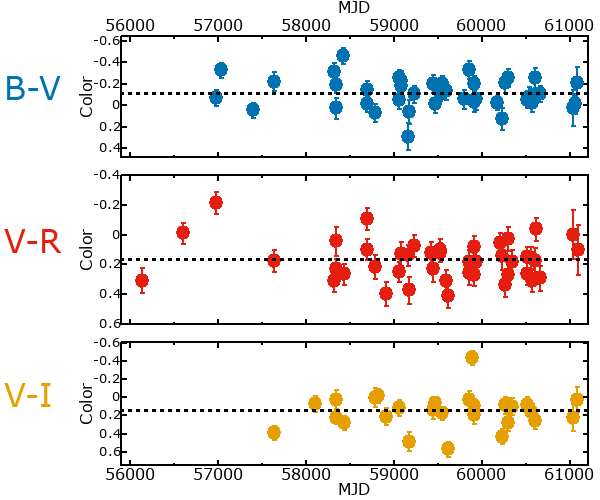## Variability

From the data available in this survey, it is not possible to tell with certainty if this star is variable.

### Correlation factors between bands

If the brightness variation is true variability then there should be a correlation between brightness changes recorded in different filter bands. The following table are the R-squared linear correlation factors calculated for the pairs of bands. The number in parentheses next to the R-squared value is the number of elements used to calculate the correlation factor. The number in square brackets next to the band is the range of magnitudes measured.

V [0.42 mag]B [0.44 mag]R [0.77 mag]
B [0.42 mag]0.30 (21)
R [0.77 mag]0.14 (23)0.24 (15)
I [0.51 mag]0.55 (15)0.40 (7)0.53 (12)

### Magnitude-Magnitude Plots

The plots below are provided to check the values in the table above and also to show any non-linear correlations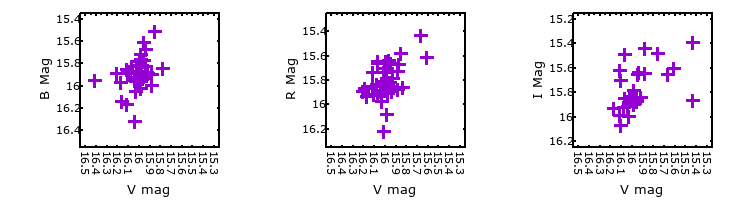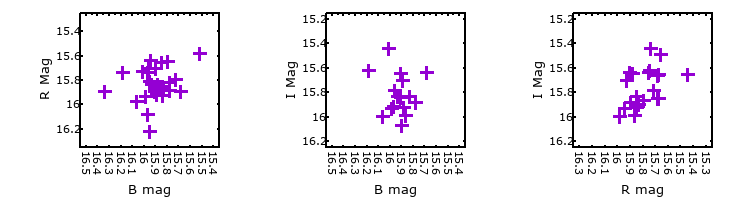### I & R Statistics

Welch & Stetson (1993, AJ, 105, 1813) present a method for varible star detection in CCD images from automated surveys. Data from two bands are compared and two statistics are calculated: R and I.

The I-statistic has an expectation value of zero for stars that are NOT variable. A larger non-zero I-value indicates a higher likelihood that the star is variable. The R statistic indicates how the color of the star changes as the variable grows brighter with R = 1.0 being no change in color.

R and I values have only been calculated if there are more than four (4) matched measurements of brightness in each band.
V
(I|R)
B
(I|R)
R
(I|R)
B
(I|R)
2.07 | 0.83
R
(I|R)
1.59 | 0.981.51 | 0.94
I
(I|R)
4.81 | 1.441.92 | 1.144.95 | 1.40